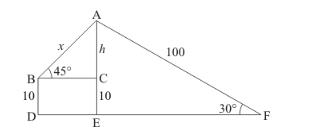# A boy is standing on the ground and flying a kite with 100 m of string at an elevation of 30°.

Question:

A boy is standing on the ground and flying a kite with 100 m of string at an elevation of 30°. Another boy is sanding on the roof of a 10 m high building and is flying his kite at an elevation of 45°. Both the boys are on opposite sides of both the kites. Find the length of the string that the second boy must have so that the two kites meet.

Solution:

Letbe the string of stringm. letbe the ground and a boy flying kite ofm string at an elevation of.And another boy flying kite of 10 m high building at an angle of elevation.Let $A E=H, A C=h, C E=10, A B=x$, and $A F=100 \mathrm{~m} . \angle A B C=45^{\circ}, \angle A F E=30^{\circ}$

Here we have to find length of string.

We use trigonometric ratios.

In ΔAFE,

$\Rightarrow \sin 30^{\circ}=\frac{A E}{A F}$

$\Rightarrow \frac{1}{2}=\frac{H}{100}$

$\Rightarrow H=50$

$\Rightarrow h=H-10$

$\Rightarrow h=50-10$

$\Rightarrow h=40$

Again in ΔABC,

$\Rightarrow \sin 45^{\circ}=\frac{A B}{A C}$

$\Rightarrow \frac{1}{\sqrt{2}}=\frac{h}{x}$

$\Rightarrow \frac{1}{\sqrt{2}}=\frac{40}{x}$

$\Rightarrow x=40 \sqrt{2}$

Hence the length of string is $40 \sqrt{2}$.